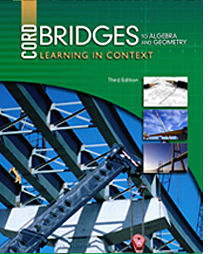# Bridges to Algebra and Geometry - 3rd Edition

## Chapter 1: Decimals and Problem Solving

Some links are repeated for use with more than one lesson.

### 1.1 The Decimal System

http://www.math.com/school/subject1/lessons/S1U1L2GL.html
Decimals and Place ValueThis site shows place values.

### 1.2 Rounding

http://www.math.com/school/subject1/lessons/S1U1L2GL.html
Decimals and Place ValueThis site shows place values.

http://www.aplusmath.com/Flashcards/rounding.html
This site has practice problems on rounding numbers.

This site has practice problems on rounding numbers.

### 1.3 Variables and Expressions

http://www.mathsisfun.com/operation-order-pemdas.html
This site presents the order of operations used when evaluating algebraic expressions, and links to some practice problems.

http://www.rootmath.org/algebra/commutative-and-associative-property
This site presents brief video tutorials on the commutative and associative properties.

http://www.rootmath.org/algebra/subtraction
This site presents brief video tutorials showing how many of the familiar properties do not apply to subtraction.

http://www.explorelearning.com/index.cfm?method=cResource.dspResourcesForCourse&CourseID=211
This site has examples and problems for the students to solve that involve adding, subtracting, and comparing decimals.

http://www.math.com/school/subject1/lessons/S1U1L4GL.html
This site shows how to add and subtract decimals and gives the rules.

### 1.5 Estimating Sums and Differences

http://www.mathsisfun.com/numbers/estimation.html
This site gives some useful guidelines for how and why to estimate, and a link to some helpful tips and tricks for estimating.

http://www.explorelearning.com/index.cfm?method=cResource.dspResourcesForCourse&CourseID=211
This site has examples and problems for the students to solve that involve adding, subtracting, and comparing decimals.

http://www.math.com/school/subject1/lessons/S1U1L4GL.html
This site shows how to add and subtract decimals and gives the rules.

This site has practice problems on subtracting with decimal numbers.

### 1.6 Properties of Multiplication

http://www.purplemath.com/modules/numbprop.htm
This site presents and practices the commutative, associative, and distributive properties.

http://www.explorelearning.com/index.cfm?method=cResource.dspResourcesForCourse&CourseID=211
This site has examples and problems for the students to solve that involve adding, subtracting, and comparing decimals.

http://www.math.com/school/subject1/lessons/S1U1L5GL.html
This site shows how to multiply decimals and gives the rules.

http://www.mathsisfun.com/numbers/math-trainer-multiply.html
This site has a multiplication trainer, where you can measure and improve your speed and accuracy at multiplying numbers.

### 1.7 Estimating Products and Quotient

http://www.explorelearning.com/index.cfm?method=cResource.dspResourcesForCourse&CourseID=211
This site has examples and problems for the students to solve that involve adding, subtracting, and comparing decimals.

http://www.math.com/school/subject1/lessons/S1U1L5GL.html
This site shows how to multiply decimals and gives the rules.

http://www.math.com/school/subject1/lessons/S1U1L6GL.html
This site shows how to divide decimals and gives the rules.

http://www.math.com/school/subject1/lessons/S1U1L6GL.html
This site shows how to divide decimals and gives the rules.

### 1.8 A Problem-Solving Strategy

http://www.math.wichita.edu/history/men/polya.html
Read about George Polya, a famous mathematician credited with developing and promoting the four-step problem solving strategy presented in the text.